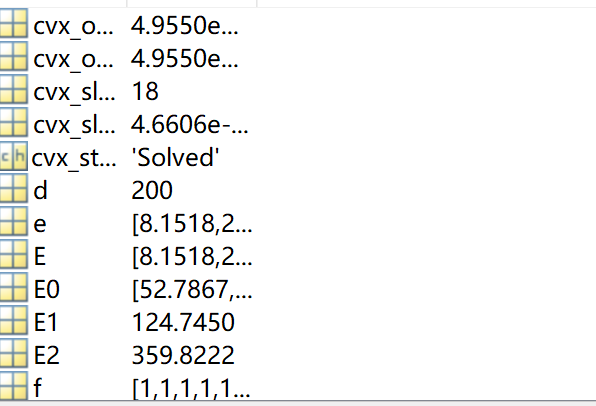# Variable value, ask for directions

I don’t know how to use the optimized variable value after CVX optimization in the next operation. Is it necessary to assign a value, or is it automatically assigned to the optimization variable. After a successful CVX optimization, my code still uses the initial value of the optimization variable.

cvx_clear
cvx_begin
variable p(6) nonnegative;%非负
variable m(6,6) nonnegative;%非负
Obj = 0;
expression m1(6)
expression m2(6)
for j=1:6
for x=1:6
if x~=j
m1(j)=m1(j)+m(j,x);
m2(j)=m2(j)+m(x,j);
end
end
Obj = Obj + (F(j)+V)/r*(3r-3B(j)-log(1+p(j)L/(3q))/log(2))-3F(j)c +(E(j)+V)p(j)+E(j)e(j)+(V(1-n)-E(j))m1(j)+nE(j)m2(j);
end
minimize(Obj)
subject to
for j=1:1:6
p(j) <=E(j)+n
m2(j)-m1(j);
end
cvx_end
m3=[0,0,0,0,0,0];
m4=[0,0,0,0,0,0];
for j=1:6
for x=1:6
if x~=j
m3(j)=m3(j)+m(j,x);
m4(j)=m4(j)+m(x,j);
end
end
f(j)=1-log2(1+p(j)L/(3q))/(3
r)-B(j)/r;
B(j)=B(j)-r
(1-f(j))+log2(1+p(j)L/(3q))/3;
F(j)= F(j)+(f(j)-c);
E(j)=E(j)-p(j)+e(j)-m3(j)+n
m4(j) ;
end

CVX can be completed, and the optimization variables are updatedHowever, the initial value of the optimization variable p(j) is used to calculate the value of f. how to solve this problem hereif CVX completes successfully, all CVX variables are at their optimal value after CVX concludes.

However, this is not necessarily so for CVX expressions, which is anything other than something declared with a variable statement. This includes declared expressions, sch as `m1` and `m2`. it also includes undeclared expressions, such as `f`,`B`,`F`,`E`. In order to ensure you are using the optimal values of CVX expressions, you must calculate them after CVX concludes, starting from declared CVX variables.

Note that, in contrast, YALMIP automatically ensures expressions are at their optimal value if the `value` command is used after optimization successfully concludes.The YALMIP behavior is nicer and avoids confusion. If I had a dollar for every time someone fell victim to this CVX “quirk”, I wouldn’t be rich, but I wouldn’t be poor.

Hello, in my question, P (J) and m (I, J) are optimization variables. When calculating f (J), only p (J) is used, but the optimized value is not used. Is there a solution here

The program’s only CVX variables are `p` and `m`. Only they are guaranteed to be at their optimal value after CVX successfully concludes. Everything else is an expression, for which the optimal value can only be known by computing them starting from CVX variables.

Internally, CVX correctly accounts for expressions when performing the optimization. So the preceding only pertains to finding out what the optimal value of the expressions are.after CVX concludes.

aftercvx_end,Shouldn’t p(j) be the optimal value? Sorry, I still don’t understand.
Only p (J) is used to calculate f,but the optimized P (J) is not used，why?

After CVX successfully concludes, `p` should have the optimal value. if you have evidence to the contrary, please provide it.

As for why CVX was designed as it is, don’t ask me, I am not the designer or developer.